Function Repository Resource:

# Exspheres

Find the exspheres of a triangle or tetrahedron

Contributed by: Minh Trinh Xuan, Ed Pegg Jr and Jan Mangaldan
 ResourceFunction["Exspheres"][{p1,p2,p3}] constructs the excircles of the triangle defined by vertices p1,p2, and p3. ResourceFunction["Exspheres"][{p1,p2,p3,p4}] constructs the exspheres of the tetrahedron defined by vertices p1,p2,p3 and p4.

## Details

An excircle is also called an escribed circle. An exsphere is also called an escribed sphere.
An incircle is internally tangent to the edges of a triangle. A triangle has three excircles that are externally tangent to each side and to the other sides when extended indefinitely.
An insphere is internally tangent to the faces of a tetrahedron. A tetrahedron has four exspheres that are externally tangent to each face at its incenter and to the other faces when extended indefinitely.
ResourceFunction["Exspheres"][poly] where poly is a Triangle or Polygon is equivalent to ResourceFunction["Exspheres"][PolygonCoordinates[poly]].
ResourceFunction["Exspheres"][poly] where poly is a Tetrahedron is equivalent to ResourceFunction["Exspheres"][PolyhedronCoordinates[poly]].
The mix of 2D and 3D for Circle and Sphere follows from Insphere and Circumsphere.

## Examples

### Basic Examples (2)

Find the excircles for a triangle:

 In:=Out=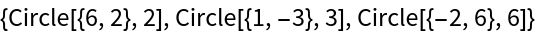Show the excircles, incircle and infinite lines:

 In:=Out=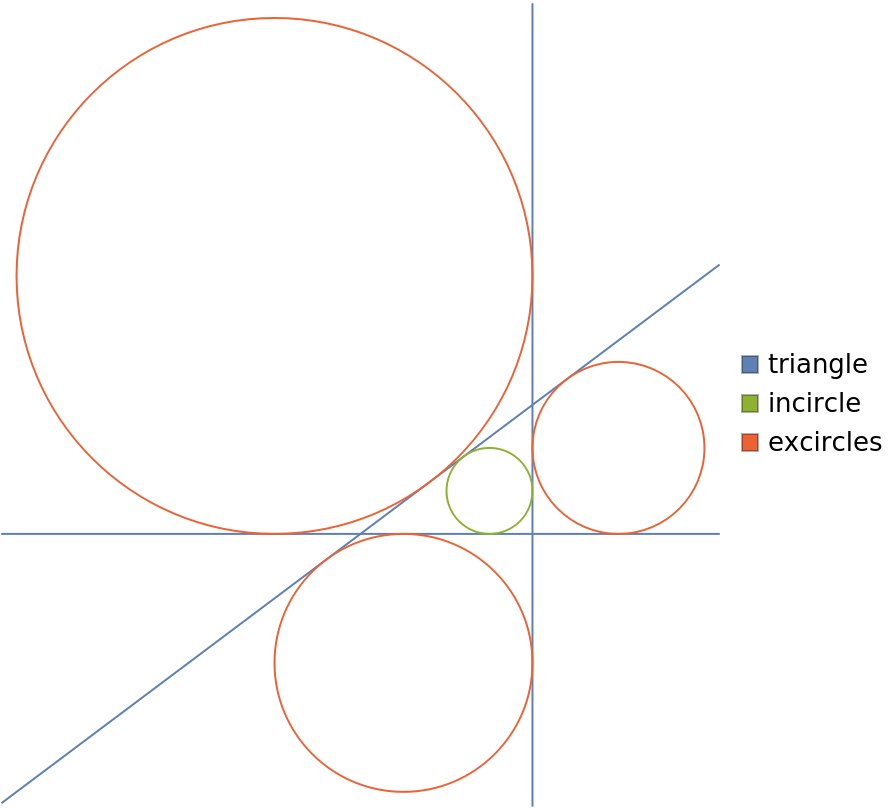### Scope (3)

A triangle:

 In:=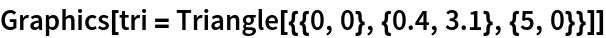Out=Show the triangle along with its excircles:

 In:=Out=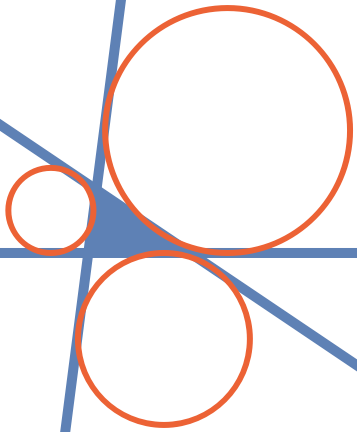Show a tetrahedron along with its exspheres:

 In:=Out=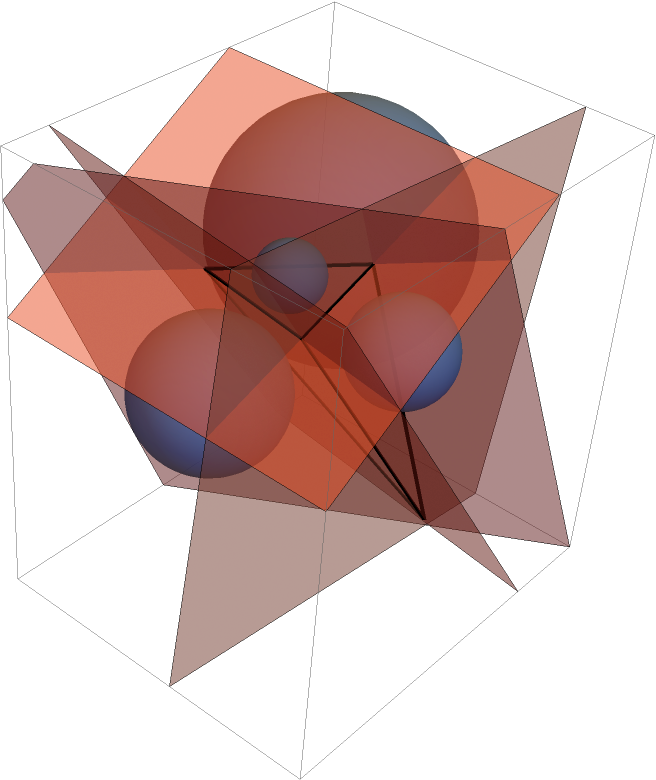Show the insphere and exspheres of a regular tetrahedron:

 In:=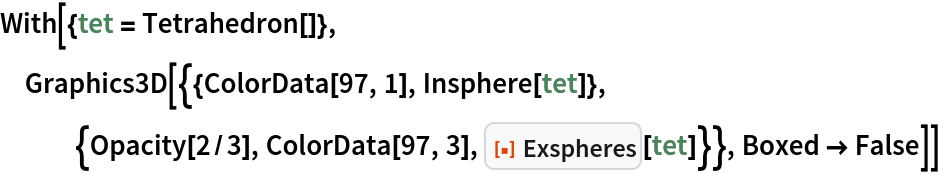Out=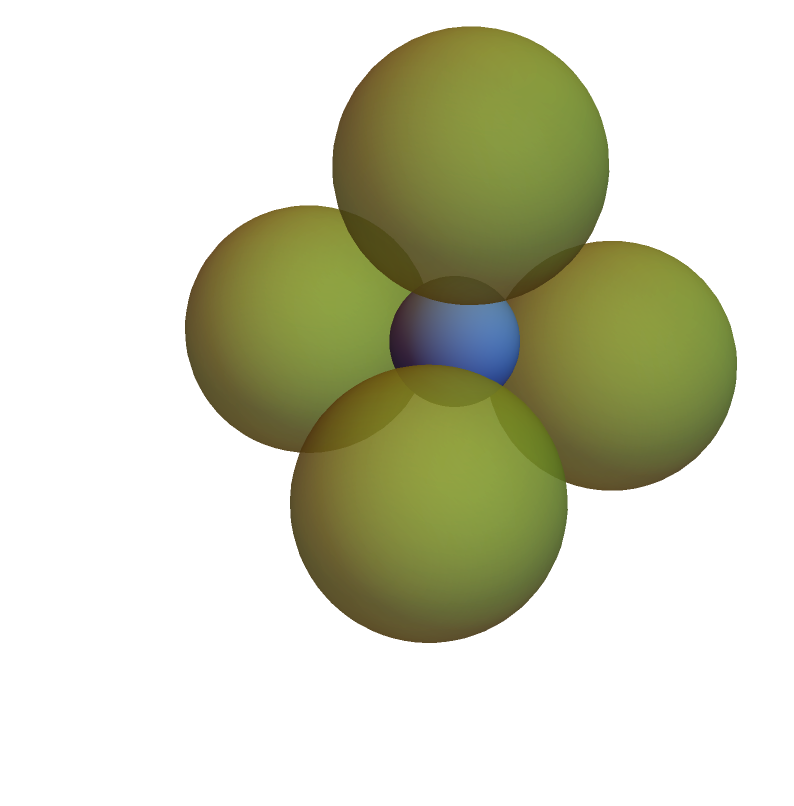### Neat Examples (2)

Show the excircles of an arbitrary triangle:

 In:=Out=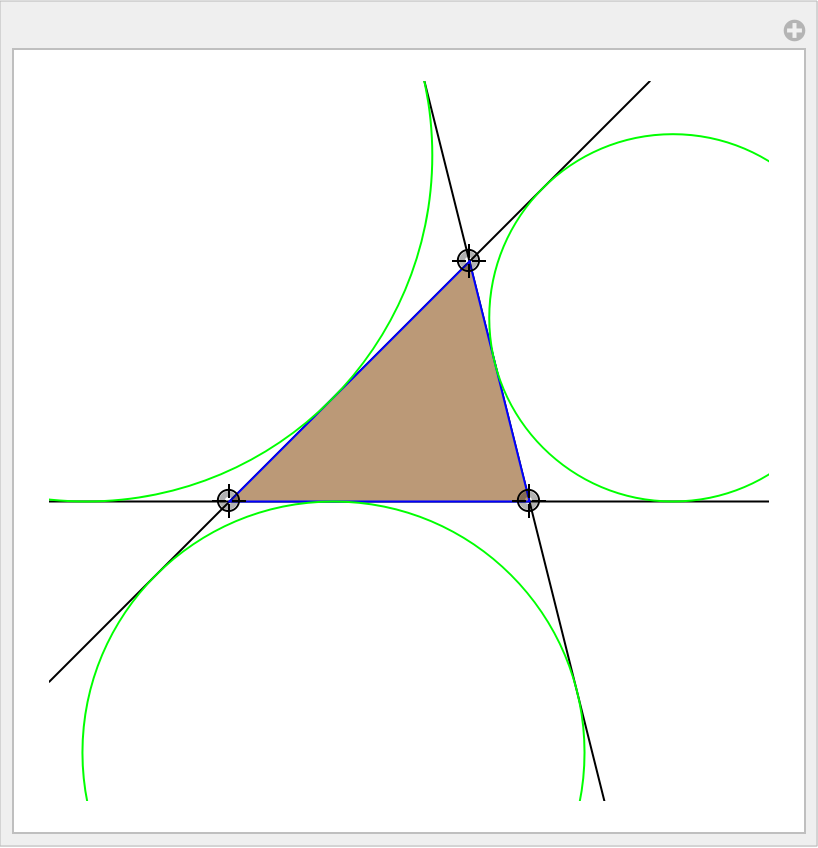A triangle and its excircles:

 In:=Out=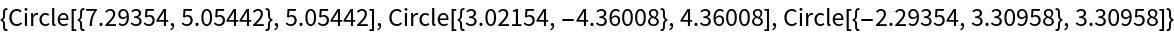Compute the center and the radius of the radical circle of the excircles:

 In:=Show the triangle, its excircles and the radical circle altogether:

 In:=Out=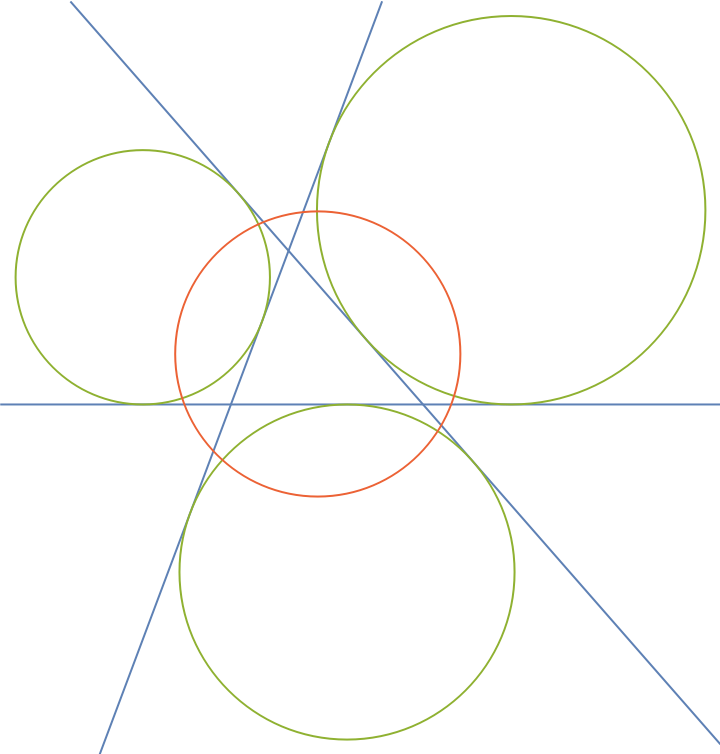## Version History

• 1.0.0 – 11 July 2022